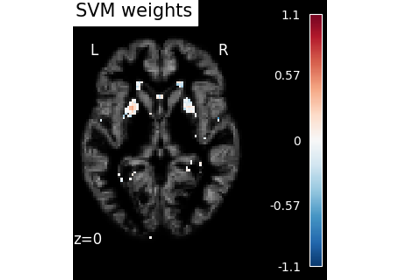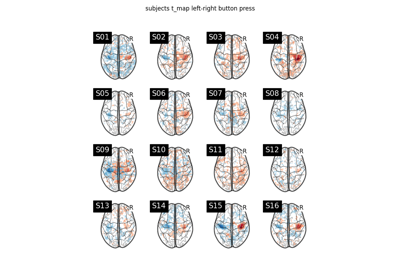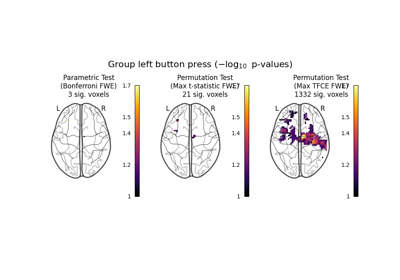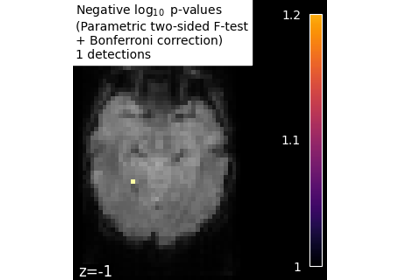Note

This page is a reference documentation. It only explains the function signature, and not how to use it. Please refer to the user guide for the big picture.

# nilearn.mass_univariate.permuted_ols#

nilearn.mass_univariate.permuted_ols(tested_vars, target_vars, confounding_vars=None, model_intercept=True, n_perm=10000, two_sided_test=True, random_state=None, n_jobs=1, verbose=0, masker=None, tfce=False, threshold=None, output_type='legacy')[source]#

Massively univariate group analysis with permuted OLS.

Tested variates are independently fitted to target variates descriptors (e.g. brain imaging signal) according to a linear model solved with an Ordinary Least Squares criterion. Confounding variates may be included in the model. Permutation testing is used to assess the significance of the relationship between the tested variates and the target variates , . A max-type procedure is used to obtain family-wise corrected p-values based on t-statistics (voxel-level FWE), cluster sizes, cluster masses, and TFCE values.

The specific permutation scheme implemented here is the one of Freedman and Lane. Its has been demonstrated in Anderson and Robinson that this scheme conveys more sensitivity than alternative schemes. This holds for neuroimaging applications, as discussed in details in Winkler et al..

Permutations are performed on parallel computing units. Each of them performs a fraction of permutations on the whole dataset. Thus, the max t-score amongst data descriptors can be computed directly, which avoids storing all the computed t-scores.

The variates should be given C-contiguous. `target_vars` are fortran-ordered automatically to speed-up computations.

Parameters:
tested_varsarray-like, shape=(n_samples, n_regressors)

Explanatory variates, fitted and tested independently from each others.

target_varsarray-like, shape=(n_samples, n_descriptors)

fMRI data to analyze according to the explanatory and confounding variates.

In a group-level analysis, the samples will typically be voxels (for volumetric data) or vertices (for surface data), while the descriptors will generally be images, such as run-wise z-statistic maps.

confounding_varsarray-like, shape=(n_samples, n_covars), optional

Confounding variates (covariates), fitted but not tested. If None, no confounding variate is added to the model (except maybe a constant column according to the value of `model_intercept`).

model_intercept`bool`, optional

If True, a constant column is added to the confounding variates unless the tested variate is already the intercept or when confounding variates already contain an intercept. Default=True.

n_perm`int`, optional

Number of permutations to perform. Permutations are costly but the more are performed, the more precision one gets in the p-values estimation. If `n_perm` is set to 0, then no p-values will be estimated. Default=10000.

two_sided_test`bool`, optional

If True, performs an unsigned t-test. Both positive and negative effects are considered; the null hypothesis is that the effect is zero. If False, only positive effects are considered as relevant. The null hypothesis is that the effect is zero or negative. Default=True.

random_state`int` or None, optional

Seed for random number generator, to have the same permutations in each computing units.

n_jobs`int`, optional

Number of parallel workers. If -1 is provided, all CPUs are used. A negative number indicates that all the CPUs except (abs(n_jobs) - 1) ones will be used. Default=1.

verbose`int`, optional

verbosity level (0 means no message). Default=0.

maskerNone or `NiftiMasker` or `MultiNiftiMasker`, optional

A mask to be used on the data. This is required for cluster-level inference, so it must be provided if `threshold` is not None.

New in version 0.9.2.

thresholdNone or `float`, optional

Cluster-forming threshold in p-scale. This is only used for cluster-level inference. If None, cluster-level inference will not be performed. Default=None.

Warning

Performing cluster-level inference will increase the computation time of the permutation procedure.

New in version 0.9.2.

tfce`bool`, optional

Whether to calculate TFCE as part of the permutation procedure or not. The TFCE calculation is implemented as described in Smith and Nichols. Default=False.

Warning

Performing TFCE-based inference will increase the computation time of the permutation procedure considerably. The permutations may take multiple hours, depending on how many permutations are requested and how many jobs are performed in parallel.

New in version 0.9.2.

output_type{‘legacy’, ‘dict’}, optional

Determines how outputs should be returned. The two options are:

• ‘legacy’: return a pvals, score_orig_data, and h0_fmax. This option is the default, but it is deprecated until 0.13, when the default will be changed to ‘dict’. It will be removed in 0.15.

• ‘dict’: return a dictionary containing output arrays. This option will be made the default in 0.13. Additionally, if `tfce` is True or `threshold` is not None, `output_type` will automatically be set to ‘dict’.

Deprecated since version 0.9.2: The default value for this parameter will change from ‘legacy’ to ‘dict’ in 0.13, and the parameter will be removed completely in 0.15.

New in version 0.9.2.

Returns:
pvalsarray-like, shape=(n_regressors, n_descriptors)

Negative log10 p-values associated with the significance test of the n_regressors explanatory variates against the n_descriptors target variates. Family-wise corrected p-values.

Note

This is returned if `output_type` == ‘legacy’.

Deprecated since version 0.9.2: The ‘legacy’ option for `output_type` is deprecated. The default value will change to ‘dict’ in 0.13, and the `output_type` parameter will be removed in 0.15.

score_orig_datanumpy.ndarray, shape=(n_regressors, n_descriptors)

t-statistic associated with the significance test of the n_regressors explanatory variates against the n_descriptors target variates. The ranks of the scores into the h0 distribution correspond to the p-values.

Note

This is returned if `output_type` == ‘legacy’.

Deprecated since version 0.9.2: The ‘legacy’ option for `output_type` is deprecated. The default value will change to ‘dict’ in 0.13, and the `output_type` parameter will be removed in 0.15.

h0_fmaxarray-like, shape=(n_regressors, n_perm)

Distribution of the (max) t-statistic under the null hypothesis (obtained from the permutations). Array is sorted.

Note

This is returned if `output_type` == ‘legacy’.

Deprecated since version 0.9.2: The ‘legacy’ option for `output_type` is deprecated. The default value will change to ‘dict’ in 0.13, and the `output_type` parameter will be removed in 0.15.

Changed in version 0.9.2: Return H0 for all regressors, instead of only the first one.

outputs`dict`

Output arrays, organized in a dictionary.

Note

This is returned if `output_type` == ‘dict’. This will be the default output starting in version 0.13.

New in version 0.9.2.

Here are the keys:

key

shape

description

t

(n_regressors, n_descriptors)

t-statistic associated with the significance test of the n_regressors explanatory variates against the n_descriptors target variates. The ranks of the scores into the h0 distribution correspond to the p-values.

logp_max_t

(n_regressors, n_descriptors)

Negative log10 p-values associated with the significance test of the n_regressors explanatory variates against the n_descriptors target variates. Family-wise corrected p-values, based on `h0_max_t`.

h0_max_t

(n_regressors, n_perm)

Distribution of the max t-statistic under the null hypothesis (obtained from the permutations). Array is sorted.

tfce

(n_regressors, n_descriptors)

TFCE values associated with the significance test of the n_regressors explanatory variates against the n_descriptors target variates. The ranks of the scores into the h0 distribution correspond to the TFCE p-values.

logp_max_tfce

(n_regressors, n_descriptors)

Negative log10 p-values associated with the significance test of the n_regressors explanatory variates against the n_descriptors target variates. Family-wise corrected p-values, based on `h0_max_tfce`.

Returned only if `tfce` is True.

h0_max_tfce

(n_regressors, n_perm)

Distribution of the max TFCE value under the null hypothesis (obtained from the permutations). Array is sorted.

Returned only if `tfce` is True.

size

(n_regressors, n_descriptors)

Cluster size values associated with the significance test of the n_regressors explanatory variates against the n_descriptors target variates. The ranks of the scores into the h0 distribution correspond to the size p-values.

Returned only if `threshold` is not None.

logp_max_size

(n_regressors, n_descriptors)

Negative log10 p-values associated with the cluster-level significance test of the n_regressors explanatory variates against the n_descriptors target variates. Family-wise corrected, cluster-level p-values, based on `h0_max_size`.

Returned only if `threshold` is not None.

h0_max_size

(n_regressors, n_perm)

Distribution of the max cluster size value under the null hypothesis (obtained from the permutations). Array is sorted.

Returned only if `threshold` is not None.

mass

(n_regressors, n_descriptors)

Cluster mass values associated with the significance test of the n_regressors explanatory variates against the n_descriptors target variates. The ranks of the scores into the h0 distribution correspond to the mass p-values.

Returned only if `threshold` is not None.

logp_max_mass

(n_regressors, n_descriptors)

Negative log10 p-values associated with the cluster-level significance test of the n_regressors explanatory variates against the n_descriptors target variates. Family-wise corrected, cluster-level p-values, based on `h0_max_mass`.

Returned only if `threshold` is not None.

h0_max_mass

(n_regressors, n_perm)

Distribution of the max cluster mass value under the null hypothesis (obtained from the permutations). Array is sorted.

Returned only if `threshold` is not None.

References

## Examples using `nilearn.mass_univariate.permuted_ols`#Voxel-Based Morphometry on Oasis dataset

Voxel-Based Morphometry on Oasis datasetSecond-level fMRI model: one sample test

Second-level fMRI model: one sample testMassively univariate analysis of a motor task from the Localizer dataset

Massively univariate analysis of a motor task from the Localizer datasetMassively univariate analysis of face vs house recognition

Massively univariate analysis of face vs house recognition